Next: The effective Skyrme force Up: The Skyrme Hartree-Fock-Bogolyubov equations Previous: Asymptotic properties of the

## Pairing correlations and divergence of the energy

The use of a zero range interaction in the particle-particle channel leads to the energy divergence problem [9,19] related to the fact that the short-range behaviour of the abnormal density is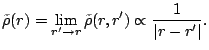(37)

The other abnormal densities diverge as well, but along that the pairing correlations also induce the divergence of the kinetic density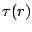. One simple way to overcome the divergence of the energy is to introduce a cut-off energy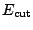in the summations of all the densities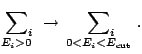(38)

This cut-off somehow simulates the finite range of the interaction in the pairing channel. It therefore constitutes an additional parameter of the interaction and must be adjusted along with the other quantities which define the pairing interaction, see e.g. Ref. .

A more elegant way to prevent the divergence was recently proposed in Refs. [9,19]. It is based on the subtractions of the divergent part of the abnormal density. In its present implementation in the code HFBRAD, this method can only be applied if the pairing force does not contains non-local terms (i.e. gradient terms). In that case, the pairing energy depends only on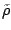(and possibly on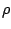but not on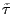or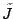), i.e.,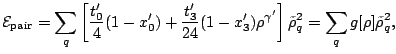(39)

and the pairing potential depends linearly on,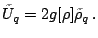(40)

The regularized pairing field can then be written as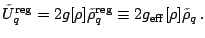(41)

Calculation of the various densities is performed by summing up the quasiparticle contributions up to a maximum energy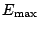, but because of the regularization, this maximum energy has not the meaning of a cut-off energy.

It can be shown  that when we evaluate the total energy of the system, the pairing energy must be defined as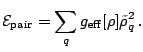(42)

With this definition, the kinetic and pairing energies both diverge but the sum, and indeed the total energy, does not . If the pairing energy density involves kinetic or gradient terms (like it was implemented for the Skyrme interaction SkP ), the regularization would require the subtraction of higher order terms. This possibility in not implemented in the present version of the code. Finally, let us note that relations (21)-(26) in Ref.  imply that the variation of quantities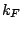and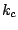with respect to the densities should be included in the mean fields. This contribution is supposed to be small and is not taken into account in the code. On the other hand, for density-dependent pairing interaction, the variation with respect to the explicit density dependence is taken into account in the code, and gives a contribution to the energy of the order of a few keV.Next: The effective Skyrme force Up: The Skyrme Hartree-Fock-Bogolyubov equations Previous: Asymptotic properties of the
Jacek Dobaczewski 2005-01-23